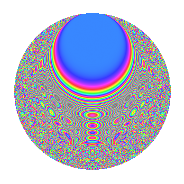# Properties

 Label 525.4.tLevel $525$ Weight $4$ Character orbit 525.t Rep. character $\chi_{525}(26,\cdot)$ Character field $\Q(\zeta_{6})$ Dimension $292$ Sturm bound $320$

# Related objects

## Defining parameters

 Level: $$N$$ $$=$$ $$525 = 3 \cdot 5^{2} \cdot 7$$ Weight: $$k$$ $$=$$ $$4$$ Character orbit: $$[\chi]$$ $$=$$ 525.t (of order $$6$$ and degree $$2$$) Character conductor: $$\operatorname{cond}(\chi)$$ $$=$$ $$21$$ Character field: $$\Q(\zeta_{6})$$ Sturm bound: $$320$$

## Dimensions

The following table gives the dimensions of various subspaces of $$M_{4}(525, [\chi])$$.

Total New Old
Modular forms 504 316 188
Cusp forms 456 292 164
Eisenstein series 48 24 24

## Trace form

 $$292q + 3q^{3} + 562q^{4} - 36q^{7} + 3q^{9} + O(q^{10})$$ $$292q + 3q^{3} + 562q^{4} - 36q^{7} + 3q^{9} - 138q^{12} - 1950q^{16} + 116q^{18} + 420q^{19} - 165q^{21} - 140q^{22} - 90q^{24} - 98q^{28} - 114q^{31} + 111q^{33} + 1044q^{36} + 552q^{37} + 954q^{39} - 1068q^{42} + 348q^{43} + 824q^{46} - 1204q^{49} - 183q^{51} - 3756q^{52} + 252q^{54} + 1362q^{57} - 710q^{58} + 2778q^{61} + 361q^{63} - 10548q^{64} + 162q^{66} + 1804q^{67} - 2258q^{72} + 2148q^{73} - 504q^{78} - 614q^{79} - 801q^{81} + 792q^{82} + 1374q^{84} + 5118q^{87} - 2782q^{88} - 838q^{91} + 2343q^{93} + 8376q^{94} - 2700q^{96} - 3006q^{99} + O(q^{100})$$

## Decomposition of $$S_{4}^{\mathrm{new}}(525, [\chi])$$ into newform subspaces

The newforms in this space have not yet been added to the LMFDB.

## Decomposition of $$S_{4}^{\mathrm{old}}(525, [\chi])$$ into lower level spaces

$$S_{4}^{\mathrm{old}}(525, [\chi]) \cong$$ $$S_{4}^{\mathrm{new}}(21, [\chi])$$$$^{\oplus 3}$$$$\oplus$$$$S_{4}^{\mathrm{new}}(105, [\chi])$$$$^{\oplus 2}$$

## Hecke characteristic polynomials

There are no characteristic polynomials of Hecke operators in the database